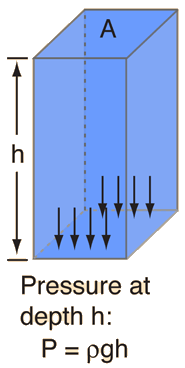# PHY C5: Density & Pressure

Here’s what we’re covering:

• Density
• Pressure
• Relationship between density & pressure

Let’s dive in!

What is Density?
Mass per unit volume of a substance.
It is denoted by the letter ρ (rho).

ρ = mass / volume
ρ = m/V

In units,
kg m-3

Solving density problems
There may be different situations involving density that may be a little challenging.
For example, mixing 2 different fluids with different densities.
What if you want to find the final mixture’s density?
Or mass?
Or the proportion of each fluid in the final mixture?

To solve these problems, make sure you understand these basic concepts:

 Each fluid has a separate density, with a separate mass & volume ρ1 = m1/V1 ρ2 = m2/V2 The TOTAL density of a mixture is the TOTAL mass divided by the TOTAL volume ρt = (m1 + m2)/(V1 + V2) You CANNOT simply add the densities together ρt ≠ m1/V1 + m2/V2 To find proportions by mass or volume, divide the wanted fluid’s mass or volume by the TOTAL mass or volume Proportion by mass of Fluid 1 = m1/(m1 + m2)Proportion by Volume of Fluid 1 = V1/(V1 + V2)

What is Pressure?
Normal (perpendicular) force acting per unit area.

P = F/A

In units,
N m-2
OR
Pa (Pascals)

Pressure can be calculated as long as you know the PERPENDICULAR component of a force acting upon an AREA.

Here’s a few different situations, with examples on how to calculate the pressure.

 An object ‘A’ resting on a surface B P = weight of A / base area of A A hammer striking the head of a nail P = Force exerted by hammer / area of nail’s head A submarine at a certain depth of water P = density of water x grav. acceleration x depth (we’ll see why later) A balloon in the atmosphere P = density of air x grav. acceleration x height of air column above

As you can see, the situations involving fluid pressure have a different formula.

Fluid Pressure
Fluid pressure can be calculated by multiplying:

• The density of the fluid (ρ)
• The depth of fluid (h)
• Gravitational acceleration (g)

P = hρg

Why?
Pressure is the WEIGHT of a column of fluid divided by the BASE AREA of that column of fluid.

Consider a column of fluid with depth h, & base area A.The volume (V) of the fluid = hA.
Weight (W) of the fluid = mass x g = Vρg
Pressure (P) at the base = W/A = Vρg/A = hρg

Fluids have some interesting properties regarding pressure:

Characteristics of Fluid Pressure

 Does NOT depend on base area of container.Does NOT depend on surface area of an object immersed inside. However, the FORCE exerted by the pressure DOES depend on the surface area. Is EQUAL at the same depth. Is exerted in ALL directions.Q: Why doesn’t the surface area of a submerged object matter?
A: When considering the pressure exerted upon the surface of an OBJECT in a fluid,
remember that the area is the TOP surface area of that object, where the fluid’s weight acts upon.
The pressure is the SAME at the same depth, no matter how large the object is.
This is because: as the AREA of the object changes, the VOLUME of the column of water above it changes proportionally.
An object with a large surface area experiences the same pressure as an object with a small surface area when submerged at the SAME DEPTH.
The larger object has a LARGER fluid column above it.
The smaller object has a SMALLER fluid column above it.
In the end, both cancel out.